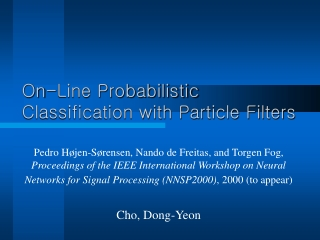# On-Line Probabilistic Classification with Particle Filters - PowerPoint PPT PresentationDownload PresentationOn-Line Probabilistic Classification with Particle Filters

On-Line Probabilistic Classification with Particle Filters
Download Presentation## On-Line Probabilistic Classification with Particle Filters

- - - - - - - - - - - - - - - - - - - - - - - - - - - E N D - - - - - - - - - - - - - - - - - - - - - - - - - - -
##### Presentation Transcript

1. On-Line Probabilistic Classification with Particle Filters Pedro Højen-Sørensen, Nando de Freitas, and Torgen Fog, Proceedings of the IEEE International Workshop on Neural Networks for Signal Processing (NNSP2000), 2000 (to appear) Cho, Dong-Yeon

2. Introduction • Sequential Classification Problems • Condition monitoring and real-time decision systems • Monitoring patients, fault detection problem • Particle filter provide an efficient and elegant probabilistic solution to this problem. • It becomes possible to compute the probabilities of class membership when the classes overlap and evolve with time. • This classification framework applied to any type of classifier, but for demonstration purposes, multi-layer perceptrons (MLPs) are used.

3. Model Specification • Markov, Nonlinear, State Space Representation • Transition model: p(t|t–1) • t Rn corresponds to the parameters (weights) of a neural network f(xt, t) • The parameters are assumed to follow a random walk t = t–1 + ut. • The process noise could be Gaussian ut ~ N(0, t2In) • Observation model: p(yt|xt,t) • xt Rnx denotes the input data at time t. • yt {0,1}ny represents the output class labels. • The likelihood of the observations should be given by the following binomial (Bernoulli) distribution

4. Estimation Objectives • Our goal will be to approximate the posterior distribution p(0: t|d1: t) and one of its marginals, the filtering density p(t|d1: t), where d1: t = {x1:t, y1:t} • By computing the filtering density recursively, we do not need to keep track of the complete history of the parameters.

5. Particle Filtering

6. Generic Particle Filter for Classification

7. Bayesian Importance Sampling Step • Importance functions • Recursive formulas • Transition prior p(t|t–1) is used as importance distribution for the MLPs.

8. Selection Step • E(Ni) = Nwt(i) • MCMC step • A skewed importance weights distribution • Many particles have no children, whereas others have a large number of children.

9. A Simple Classification Example • Experimental Setup • An MLP with 4 hidden logistic functions and an output logistic function • N = 200, t = 0.2 (0 = 10)

10. Results

11. An Application to Fault Detection • Monitoring the exhaust valve condition in a marine diesel engine • The main goal • Detection of the leakage before the engine performance becomes unacceptable or irreversible damage occurs.

12. Experimental Setup and Results • An MLP with 2 hidden unit and 5 input nodes (PCA is used for dimensionality reduction.) • 500 particles

13. Conclusions • We presented a novel on-line classification scheme and demonstrated it on two problems. • We believe this strategy has great potential and that it needs to be further tested on other types of parametric classifiers and classification domains.